# Drawing Hexagons

## Introduction

Many tabletop role playing games use a map drawn on hexagons to show where players and other objects are. They do this because hexagons have an equal distance from center to center, any way you go, where squares do not.

Unfortunately, drawing squares on the screen is lots easier than drawing hexagons. Hit-detection and positioning are simpler too, so most computer programs wind up using squares, or at the least, rectangles. While this is perfectly acceptable, it's not as effective, and offers a different gaming experience.

There are many interesting resources on the web to handle gaming. Some of them talk about using hexes, but don't talk about how to actually draw them, or how to do anything useful like hit testing. Probably, if you're not as math-impaired as I am, this is a straightforward thing to do, and you'll have no trouble. However, it took me some doing, and I thought I'd write it up here so that someone else might not have to do the same.

### Representing Hexes

There are several suggestions on how to store the contents of hexagons on a web page I can't find the URL to at the moment. I found most of them more complex than need be, and discovered that storing the contents of the hexes in a two dimensional array worked best. A grid of hexes really is just grid, but with a row offset by half a hex. Storing this is much simpler than the other suggestions I read.

My friend tangaloor helped a lot in getting my brain around this. He and I developed a quick way to draw hexes which wasn't quite spot on, and drew irregular hexes. The thinking I did there led me here.

## Drawing Hexes

### How I Figured It Out

Drawing a real, regular hexagon baffled me for a long time. I finally sat down with some graph paper, some pencils, and some hex paper and started making scribbly notes. What I finally realized, is that a regular hexagon could be broken up in to a rectangle, and four right triangles. Once I knew this, it came together pretty well.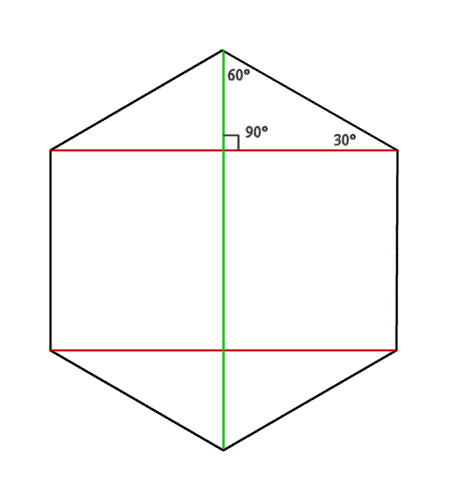This hex shows the triangles I'm talking about. The angles are reasonably easily fathomed; each interior angle of a regular hexagon is a 120 degree angle, and we know that the red lines are perpendicular to the green line; it must be a 30-60-90 triangle, and identifying the 30 and 60 degree sides are pretty straightforward.

Once you've broken the hex in to nice triangles, it becomes a little easier how to see the things you want to know. In a regular hexagon, all the sides are the same length. Extracting one triangle, and labeling the sides gives us some names to work with.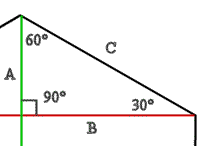If you look at the full hex above, you'll see that if you know the lengths of all three sides of this triangle, you can plot points on a grid to draw a hexagon pretty easily. And, since we know one of the lengths of the sides, we can get the rest through some good old fashioned trigonometry.

We need to know A, B, and C. We are given C.

We can calculate side A from this: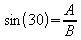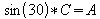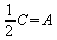That's a pretty reasonable formula.

The other is similar, but doesn't boil down to such nice clear numbers: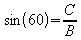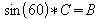Sin 60 is a constant, though, which can be evaluated, or stored as a constant (0.866) in your program to whatever degree of accuracy you feel the need to use.

That is: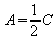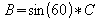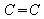(given)

## Drawing a Hex

To actually draw a hex, you need to figure out the points for each point of the hex. There are two ways of drawing a hex, one, called North-South, has the parallel edges running vertically on the page. The other, called East-West has the parallel edges running horizontally. Using the lengths above, it becomes pretty straightforward to calculate points for the hex.

I have numbered the points of the hex simply to show which is which; the numbers have no real bearing on anything.

### North-South

A North-South hex can be drawn by using the following points.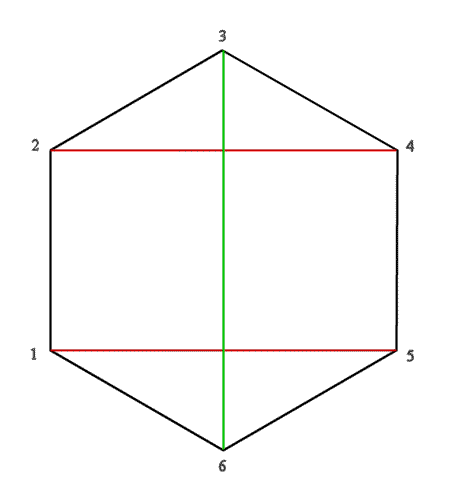Point

X Coordinate

Y Coordinate

1

0

A + C

2

0

A

3

B

0

4

2 * B

A

5

2 * B

A + C

6

B

2 * C

### East-West

An East-West hex can be drawn using the following points.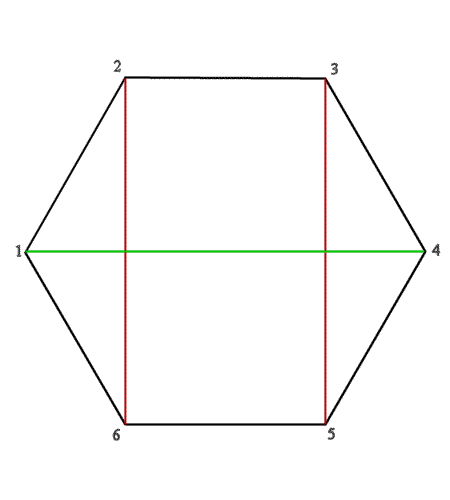Point

X Coordinate

Y Coordinate

1

0

B

2

A

0

3

A + C

0

4

2 * C

B

5

A + C

2 * B

6

A

2 * B